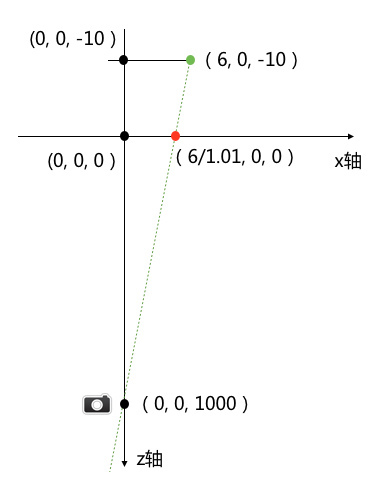0%

# 变换矩阵

$\begin{bmatrix} m11 & m12 & m13 & m14 \\ m21 & m22 & m23 & m24 \\ m31 & m32 & m33 & m34 \\ m41 & m42 & m43 & m44 \end{bmatrix}$

$\begin{bmatrix} x & y & z & 1 \end{bmatrix} \times \begin{bmatrix} m11 & m12 & m13 & m14 \\ m21 & m22 & m23 & m24 \\ m31 & m32 & m33 & m34 \\ m41 & m42 & m43 & m44 \end{bmatrix} = \\ \begin{bmatrix} m11x+m21y+m31z+m41 & m12x+m22y+m32z+m42 & m13x+m23y+m33z+m43 & m14+m24+m34+m44 \end{bmatrix}$

• 这里定义的向量最后一位表示齐次向量元素，如果不为1，需要再化为齐次坐标（通常情况可以取m14,m24,m34,m44为0,0,0,1），对应真正的x,y,z坐标

$\begin{bmatrix} \frac{m11x+m21y+m31z+m41}{m14+m24+m34+m44} & \frac{m12x+m22y+m32z+m42}{m14+m24+m34+m44} & \frac{m13x+m23y+m33z+m43}{m14+m24+m34+m44} \end{bmatrix}$

• iOS设备上，是按照左手系的三维空间，即正面面对设备屏幕，坐标原点从屏幕左上方起，x轴指向右方，y轴指向下方，z轴为屏幕指向眼球。而macOS上是右手系，原点是屏幕左下角，x轴指向右方，y轴指向上方（相反），z轴同样为屏幕指向眼球，要注意# 平移

$\begin{bmatrix} x & y & z & 1 \end{bmatrix} \times \begin{bmatrix} 1 & 0 & 0 & 0 \\ 0 & 1 & 0 & 0 \\ 0 & 0 & 1 & 0 \\ a & b & c & 1 \end{bmatrix} = \begin{bmatrix} x+a & y+b & z+c & 1 \end{bmatrix}$

# 缩放

$\begin{bmatrix} x & y & z & 1 \end{bmatrix} \times \begin{bmatrix} a & 0 & 0 & 0 \\ 0 & a & 0 & 0 \\ 0 & 0 & a & 0 \\ 0 & 0 & 0 & 1 \end{bmatrix} = \begin{bmatrix} ax & ay & az & 1 \end{bmatrix}$

# 旋转

$\begin{cases} x^{‘} = x \cos \theta - y \sin \theta \\ y^{‘} = y \cos \theta + x \sin \theta \\ z^{‘} = z \end{cases}$

$\begin{bmatrix} x & y & z & 1 \end{bmatrix} \times \begin{bmatrix} 1 & 0 & 0 & 0 \\ 0 & \cos \theta & \sin \theta & 0 \\ 0 & - \sin \theta & \cos \theta & 0 \\ 0 & 0 & 0 & 1 \end{bmatrix} = \begin{bmatrix} x & y \cos \theta - z \sin \theta & z \cos \theta + y \sin \theta& 1 \end{bmatrix}$

$\begin{bmatrix} x & y & z & 1 \end{bmatrix} \times \begin{bmatrix} \cos \theta & 0 & - \sin \theta & 0 \\ 0 & 1 & 0 & 0 \\ \sin \theta & 0 & \cos \theta & 0 \\ 0 & 0 & 0 & 1 \end{bmatrix} = \begin{bmatrix} x \cos \theta + z \sin \theta & y & z \cos \theta - x \sin \theta & 1 \end{bmatrix}$

# 错切

$\begin{bmatrix} x & y & z & 1 \end{bmatrix} \times \begin{bmatrix} 1 & m21 & 0 & 0 \\ m12 & 1 & 0 & 0 \\ 0 & 0 & 1 & 0 \\ 0 & 0 & 0 & 1 \end{bmatrix} = \begin{bmatrix} x+m12y & m21x+y & z & 1 \end{bmatrix}$

# 透视投影## 原理和推导：

Core Animation已经定义了焦点的x,y坐标，就是这个图层的anchorPoint（锚点），同时取z=0的XoY平面作为图像平面（也就是iPhone的屏幕平面），那么假如我希望投影中心到图像平面的距离是d，可以假设焦点坐标为(0,0,d)，现在对m34的值进行赋值为w，初始向量坐标为(x,y,z)，开始推导：

$\begin{bmatrix} x & y & z & 1 \end{bmatrix} \times \begin{bmatrix} 1 & 0 & 0 & 0 \\ 0 & 1 & 0 & 0 \\ 0 & 0 & 1 & w \\ 0 & 0 & 0 & 1 \end{bmatrix} = \begin{bmatrix} x & y & z & zw+1 \end{bmatrix}$

$\begin{bmatrix} x^{‘} & y^{‘} & z^{‘} \end{bmatrix} = \begin{bmatrix} \frac{x}{zw+1} & \frac{y}{zw+1} & \frac{z}{zw+1} \end{bmatrix}$$\because 由图，依据相似三角形原理 \\ \therefore \left| \frac{x}{zw+1}: x \right| = d : (\left|z\right|+d) \\ 去绝对值号，且d\neq0,x\neq0，得 \\ \frac{1}{zw+1} = \frac{1}{1-\frac{z}{d}} \\ \therefore w = - \frac{1}{d}$# 总结

Core Animation提供的CATransform3D主要的几个变换都在这里介绍了，尤其是透视投影，一定要理解原理，知道为什么需要修改m34来控制透视焦点。

# 参考资料

iOS Core Animation Techniques

iOS的三维透视投影